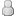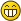• 11 Votes - 4.64 Average
• 1
• 2
• 3
• 4
• 5
11-14-2015, 06:08 PM (This post was last modified: 11-14-2015 06:12 PM by LunaMoo.)Post: #226
 LunaMooPosting FreakPosts: 1,749 Joined: Sep 2013 Reputation: 158
Heh reuploaded again, @t3nsini sorry for that - should be fixed now. I think my gpu's drivers are auto correcting common mistakes/typos or something since that was caused by something I missed when converting one filter from HLSL to GLSL. Suprising that your nvidia gpu doesn't do that, it's neither bad nor old and should be more friendly for mistakes than my amd gpu's.(Edit: I would guess that might be due to windows 10 which drivers certainly aren't as good nor polished as on other systems yet.)

Either way I hope nothing more like that is hidding there, unfortunately since it works for me due to some magic, can't be certain of that anymore.

http://forums.ppsspp.org/showthread.php?tid=3590&pid=117172#pid117172 - simple CE scripts to help creating CWCheats,
https://github.com/LunaMoo/PPSSPP_workarounds - CWCheat workarounds.
11-15-2015, 11:50 PMPost: #227
 t3nsiniJunior MemberPosts: 3 Joined: Nov 2015 Reputation: 0
LunaMoo

Worked!!! YAY!!! Thx! U´re the best!11-21-2015, 03:38 PMPost: #228
 Nick001Junior MemberPosts: 24 Joined: Aug 2015 Reputation: 0
(11-07-2015 04:16 AM)[Unknown] Wrote:
(11-07-2015 12:13 AM)LunaMoo Wrote:  Yeah about games pausing rendering for loading screens and such we probably can't do much at least not in a way it currently works.

That sounds like something we could add a flag to the post shader ini to change. Not volunteering to implement it, but it ought to be doable.

-[Unknown]

ALL the cookies to whoever would do that. Seriously all of them.

Though I do realize it would take time away from more important issues.
11-25-2015, 01:07 PMPost: #229
 dj001Junior MemberPosts: 6 Joined: Nov 2013 Reputation: 0
Hi. This is possible to disable Smooth Icons. Like item icons, sword icon near hero name... I can't disable this.
11-28-2015, 12:31 PM (This post was last modified: 11-28-2015 12:32 PM by shinra358.)Post: #230
 shinra358MemberPosts: 65 Joined: Aug 2013 Reputation: 0
Can you add the spline shader and port/replace byuu's scanlines from Higan (his is easier to configure) OR add an image overlay shader (displays image from a png and adjusts transparency)?
12-21-2015, 05:07 PMPost: #231
 eddiefurJunior MemberPosts: 14 Joined: Dec 2015 Reputation: 0
GLSL Documentation / Tutorial - Cel Shading Wind Waker
Hello everyone,

I started working on a shader that looks like the cel shading used in Zelda Wind Waker. I'm only limited to the actual codes of the shaders available with ppsspp. Those shader are kind of hard to understand because I'm new with the GLSL and those shaders use non meaningful variables and there is no comments...

I would like to know ALL variables I can used from PPSSPP to make the best shader as possible. I mean, can I have access to vertex normal, lighting or any sort of matrix? The actual shader from ppsspp use only textures and texel (I don't know what it is).

A lot of websites tell that cel shading is based on normal and lighting... and that's where I'm stucked... I tried to do my cel shading wind waker like with only textures informations and I've got some results but not as good as I expected...

Can you tell me where I can find some documentation to help me with my shader creation?

Thank you,

sorry for my language, I'm french...
12-22-2015, 05:22 PMPost: #232
 eddiefurJunior MemberPosts: 14 Joined: Dec 2015 Reputation: 0
RE: GLSL Documentation / Tutorial - Cel Shading Wind Waker
I think I've got some great results so far... there is the code :

attribute vec4 a_position;
attribute vec2 a_texcoord0;

varying vec4 v_texcoord0;

void main()
{
gl_Position = a_position;
v_texcoord0=a_texcoord0.xyxy;
}

uniform sampler2D sampler0;

varying vec4 v_texcoord0;

vec3 rgb2hsv(vec3 c)
{
vec4 K = vec4(0.0, -1.0 / 3.0, 2.0 / 3.0, -1.0);
vec4 p = mix(vec4(c.bg, K.wz), vec4(c.gb, K.xy), step(c.b, c.g));
vec4 q = mix(vec4(p.xyw, c.r), vec4(c.r, p.yzx), step(p.x, c.r));

float d = q.x - min(q.w, q.y);
float e = 1.0e-10;
return vec3(abs(q.z + (q.w - q.y) / (6.0 * d + e)), d / (q.x + e), q.x);
}

vec3 hsv2rgb(vec3 c)
{
vec4 K = vec4(1.0, 2.0 / 3.0, 1.0 / 3.0, 3.0);
vec3 p = abs(fract(c.xxx + K.xyz) * 6.0 - K.www);
return c.z * mix(K.xxx, clamp(p - K.xxx, 0.0, 1.0), c.y);
}

void main()
{
vec3 ct = texture2D(sampler0, v_texcoord0.zw).xyz;
vec3 hsv = rgb2hsv(ct);

if (hsv.z >= 0 && hsv.z < 0.05) hsv.z = 0.025;
else if (hsv.z >= 0.05 && hsv.z < 0.2) hsv.z = 0.125;
else if (hsv.z >= 0.2 && hsv.z < 0.25) hsv.z = 0.225;
else if (hsv.z >= 0.25 && hsv.z < 0.30) hsv.z = 0.275;
else if (hsv.z >= 0.30 && hsv.z < 0.45) hsv.z = 0.375;
else if (hsv.z >= 0.45 && hsv.z < 0.50) hsv.z = 0.475;
else if (hsv.z >= 0.5 && hsv.z < 0.55) hsv.z = 0.525;
else if (hsv.z >= 0.55 && hsv.z < 0.70) hsv.z = 0.625;
else if (hsv.z >= 0.70 && hsv.z < 0.75) hsv.z = 0.725;
else if (hsv.z >= 0.75 && hsv.z < 0.8) hsv.z = 0.775;
else if (hsv.z >= 0.8 && hsv.z < 0.95) hsv.z = 0.875;
else if (hsv.z >= 0.95 && hsv.z <= 1) hsv.z = 0.975;

ct = hsv2rgb(hsv);

gl_FragColor.xyz = ct;
gl_FragColor.a = 1.0;
}
12-22-2015, 08:51 PMPost: #233
 eddiefurJunior MemberPosts: 14 Joined: Dec 2015 Reputation: 0
RE: GLSL Documentation / Tutorial - Cel Shading Wind Waker

uniform sampler2D sampler0;

varying vec4 v_texcoord0;

void main()
{
vec3 ct = texture2D(sampler0, v_texcoord0.zw).xyz;
float br = max(max(ct.r, ct.g), ct.b);
float nbr;

if (br >= 0 && br < 0.05) nbr = 0.025;
else if (br >= 0.05 && br < 0.2) nbr = 0.125;
else if (br >= 0.2 && br < 0.25) nbr = 0.225;
else if (br >= 0.25 && br < 0.30) nbr = 0.275;
else if (br >= 0.30 && br < 0.45) nbr = 0.375;
else if (br >= 0.45 && br < 0.50) nbr = 0.475;
else if (br >= 0.5 && br < 0.55) nbr = 0.525;
else if (br >= 0.55 && br < 0.70) nbr = 0.625;
else if (br >= 0.70 && br < 0.75) nbr = 0.725;
else if (br >= 0.75 && br < 0.8) nbr = 0.775;
else if (br >= 0.8 && br < 0.95) nbr = 0.875;
else if (br >= 0.95 && br <= 1) nbr = 0.975;

float mul = nbr / br;
ct *= mul;

gl_FragColor.xyz = ct;
gl_FragColor.a = 1.0;
}
01-03-2016, 12:08 AMPost: #234
 eddiefurJunior MemberPosts: 14 Joined: Dec 2015 Reputation: 0
My new shader - Nintendo DS Auto Contrast like
Hello everyone, here's a shader I came with today. It's a shader inspired by subtle constrast and contouring we can see in some Nintendo DS games. It adds so much clarity in your psp game. Have fun playing gamesuniform sampler2D sampler0;
uniform vec2 u_texelDelta;

varying vec4 v_texcoord0;

void main()
{
vec3 cu = texture2D(sampler0, v_texcoord0.xy + (vec2(0.0, -1.0) * u_texelDelta)).xyz;
vec3 cd = texture2D(sampler0, v_texcoord0.xy + (vec2(0.0, 1.0) * u_texelDelta)).xyz;
vec3 cl = texture2D(sampler0, v_texcoord0.xy + (vec2(-1.0, 0.0) * u_texelDelta)).xyz;
vec3 cr = texture2D(sampler0, v_texcoord0.xy + (vec2(1.0, 0.0) * u_texelDelta)).xyz;
vec3 c = texture2D(sampler0, v_texcoord0.xy).xyz;
float lc = max(max(c.r, c.g), c.b);
float d=0;
float nd=0;

nd = max(max(cu.r-c.r, cu.g-c.g), cu.b-c.b);
if(nd > d) d=nd;
nd = max(max(cd.r-c.r, cd.g-c.g), cd.b-c.b);
if(nd > d) d=nd;
nd = max(max(cl.r-c.r, cl.g-c.g), cl.b-c.b);
if(nd > d) d=nd;
nd = max(max(cr.r-c.r, cr.g-c.g), cr.b-c.b);
if(nd > d) d=nd;

gl_FragColor.xyz = c * (lc - d) / lc;
gl_FragColor.a = 1.0;
}

attribute vec4 a_position;
attribute vec2 a_texcoord0;

varying vec4 v_texcoord0;

void main()
{
gl_Position = a_position;
v_texcoord0=a_texcoord0.xyxy;
}
01-03-2016, 09:26 AMPost: #235vnctdj>>> MHFU Fan <<<Posts: 5,388 Joined: Mar 2013 Reputation: 303
Merged.

♦ Intel Core i7-6700HQ | 16 GB RAM | NVIDIA GeForce GTX 960M | Debian Testing
♦ Intel Core i7-2630QM | 4 GB RAM | NVIDIA GeForce GT 540M | Debian Testing
♦ PSP-3004 | 6.60 PRO-C2
01-04-2016, 03:59 PM (This post was last modified: 01-05-2016 08:38 PM by eddiefur.)Post: #236
 eddiefurJunior MemberPosts: 14 Joined: Dec 2015 Reputation: 0
Very good shader from http://www.raywenderlich.com/10862/how-t...cos2d-2-x. It a MUST HAVE in PPSSPP. It adds so much depth into the games.

uniform sampler2D sampler0;
uniform vec2 u_texelDelta;
varying vec4 v_texcoord0;

void main()
{
vec3 ct = texture2D(sampler0, v_texcoord0.xy).xyz;
ct += texture2D(sampler0, v_texcoord0.xy - (vec2(1.0, 1.0) * u_texelDelta)).xyz;
ct -= texture2D(sampler0, v_texcoord0.xy + (vec2(1.0, 1.0) * u_texelDelta)).xyz;

gl_FragColor.xyz = ct;
gl_FragColor.a = 1.0;
}

attribute vec4 a_position;
attribute vec2 a_texcoord0;

varying vec4 v_texcoord0;

void main()
{
gl_Position = a_position;
v_texcoord0=a_texcoord0.xyxy;
}
01-08-2016, 04:12 PMPost: #237
 DemetriusJunior MemberPosts: 17 Joined: Nov 2014 Reputation: 0
LunaMoo, please check out your PM. Thank you01-08-2016, 10:36 PMPost: #238
 eddiefurJunior MemberPosts: 14 Joined: Dec 2015 Reputation: 0
Modification of the emboss / bump map shader. Really much better.

uniform sampler2D sampler0;
uniform vec2 u_texelDelta;
varying vec4 v_texcoord0;

vec3 emboss(vec2 textcoord, float str) {
bool ok = true;
vec3 c = texture2D(sampler0, textcoord.xy).xyz;
vec3 cp = texture2D(sampler0, textcoord.xy - (vec2(1.0, 1.0) * u_texelDelta)).xyz;
vec3 cm = texture2D(sampler0, textcoord.xy + (vec2(1.0, 1.0) * u_texelDelta)).xyz;
float l = max(max(c.r, c.g), c.b);
float nl;
while(ok && str > 0) {
nl = l;
nl += max(max(cp.r, cp.g), cp.b) * str;
nl -= max(max(cm.r, cm.g), cm.b) * str;
if(abs(nl - l) < 0.1) ok = false;
else str-=0.1;
}
if(str > 0) return c * nl / l;
else return vec3(1, 0, 0);
}

void main ()
{
gl_FragColor.rgb = emboss(v_texcoord0.xy, 2); // 2 is the strength of the effect... the higher the more strength
gl_FragColor.a = 1;
}
01-09-2016, 08:37 AMPost: #239
 GamerzHell9137Senior MemberPosts: 331 Joined: Feb 2013 Reputation: 5
(01-08-2016 10:36 PM)eddiefur Wrote:  Modification of the emboss / bump map shader. Really much better.

uniform sampler2D sampler0;
uniform vec2 u_texelDelta;
varying vec4 v_texcoord0;

vec3 emboss(vec2 textcoord, float str) {
bool ok = true;
vec3 c = texture2D(sampler0, textcoord.xy).xyz;
vec3 cp = texture2D(sampler0, textcoord.xy - (vec2(1.0, 1.0) * u_texelDelta)).xyz;
vec3 cm = texture2D(sampler0, textcoord.xy + (vec2(1.0, 1.0) * u_texelDelta)).xyz;
float l = max(max(c.r, c.g), c.b);
float nl;
while(ok && str > 0) {
nl = l;
nl += max(max(cp.r, cp.g), cp.b) * str;
nl -= max(max(cm.r, cm.g), cm.b) * str;
if(abs(nl - l) < 0.1) ok = false;
else str-=0.1;
}
if(str > 0) return c * nl / l;
else return vec3(1, 0, 0);
}

void main ()
{
gl_FragColor.rgb = emboss(v_texcoord0.xy, 2); // 2 is the strength of the effect... the higher the more strength
gl_FragColor.a = 1;
}

I doubt that anyone understands what you're talking about. If you made a new custom shader then post the file so we can use it in PPSSPP.
01-10-2016, 03:31 PMPost: #240
 eddiefurJunior MemberPosts: 14 Joined: Dec 2015 Reputation: 0